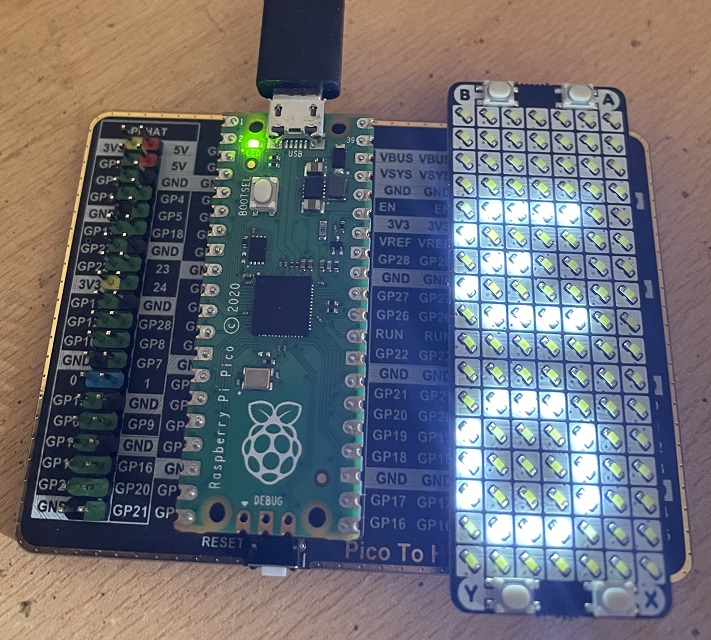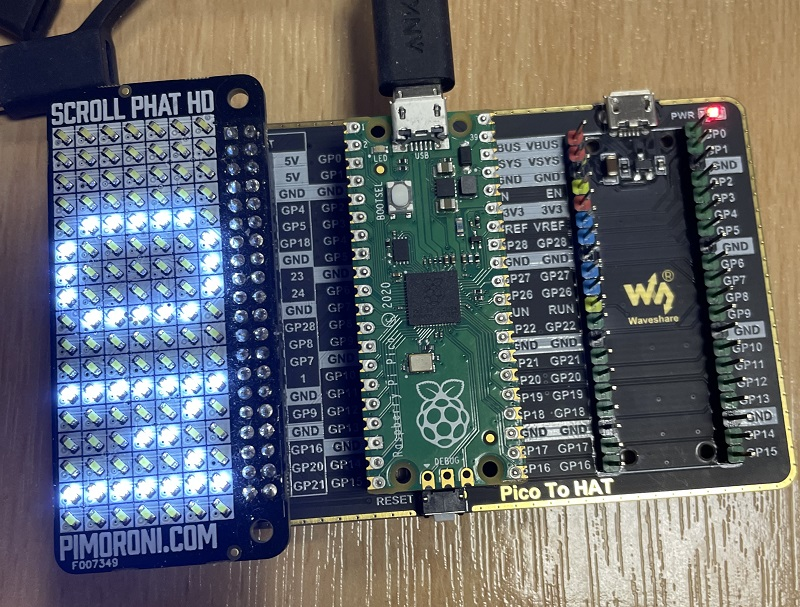# Raspberry Pi Pico Scroll Pack

The Pico Scroll Pack is a Pimoroni product. It uses the IS31FL3731 LED matrix driver chip to drive a charlieplexed 17x7 LED matrix. Pimoroni have made lots of different displays like this, for the Raspberry Pi and the micro:bit as well as a smaller 11x7 version for their 'breakout garden' range. This board also comes with 4 little switches.

In this image I am using a Pico To HAT adapter that has some spare pins that I can place the Scroll Pack onto.Pimoroni have a driver module that you can download for this. I decided to go pure MicroPython and adapt the scroll:bit MicroPython library that Pimoroni provided. This was lightweight by design to deal with the lack of memory on the micro:bit.

Save this library as is31fl3731.py. I have added some line breaks in the font definition so that it displays nicely on this page. Remove them when you use it.

```from time import sleep_ms

font = bytearray(b'\x00\x00\x00\x00\x00\x00\x00\x5f\x00\x00\x00\x07\x00\x07\x00\x14\x7f\x14
\x7f\x14\x24\x2a\x7f\x2a\x12\x23\x13\x08\x64\x62\x36\x49\x55\x22\x50\x00\x05\x03\x00\x00\x00
\x1c\x22\x41\x00\x00\x41\x22\x1c\x00\x14\x08\x3e\x08\x14\x08\x08\x3e\x08\x08\x00\x50\x30\x00
\x00\x08\x08\x08\x08\x08\x00\x60\x60\x00\x00\x20\x10\x08\x04\x02\x3e\x51\x49\x45\x3e\x00\x42
\x7f\x40\x00\x42\x61\x51\x49\x46\x21\x41\x45\x4b\x31\x18\x14\x12\x7f\x10\x27\x45\x45\x45\x39
\x3c\x4a\x49\x49\x30\x01\x71\x09\x05\x03\x36\x49\x49\x49\x36\x06\x49\x49\x29\x1e\x00\x36\x36
\x00\x00\x00\x56\x36\x00\x00\x08\x14\x22\x41\x00\x14\x14\x14\x14\x14\x00\x41\x22\x14\x08\x02
\x01\x51\x09\x06\x32\x49\x79\x41\x3e\x7e\x11\x11\x11\x7e\x7f\x49\x49\x49\x36\x3e\x41\x41\x41
\x22\x7f\x41\x41\x22\x1c\x7f\x49\x49\x49\x41\x7f\x09\x09\x09\x01\x3e\x41\x49\x49\x7a\x7f\x08
\x08\x08\x7f\x00\x41\x7f\x41\x00\x20\x40\x41\x3f\x01\x7f\x08\x14\x22\x41\x7f\x40\x40\x40\x40
\x7f\x02\x0c\x02\x7f\x7f\x04\x08\x10\x7f\x3e\x41\x41\x41\x3e\x7f\x09\x09\x09\x06\x3e\x41\x51
\x21\x5e\x7f\x09\x19\x29\x46\x46\x49\x49\x49\x31\x01\x01\x7f\x01\x01\x3f\x40\x40\x40\x3f\x1f
\x20\x40\x20\x1f\x3f\x40\x38\x40\x3f\x63\x14\x08\x14\x63\x07\x08\x70\x08\x07\x61\x51\x49\x45
\x43\x00\x7f\x41\x41\x00\x02\x04\x08\x10\x20\x00\x41\x41\x7f\x00\x04\x02\x01\x02\x04\x40\x40
\x40\x40\x40\x00\x01\x02\x04\x00\x20\x54\x54\x54\x78\x7f\x48\x44\x44\x38\x38\x44\x44\x44\x20
\x38\x44\x44\x48\x7f\x38\x54\x54\x54\x18\x08\x7e\x09\x01\x02\x0c\x52\x52\x52\x3e\x7f\x08\x04
\x04\x78\x00\x44\x7d\x40\x00\x20\x40\x44\x3d\x00\x7f\x10\x28\x44\x00\x00\x41\x7f\x40\x00\x7c
\x04\x18\x04\x78\x7c\x08\x04\x04\x78\x38\x44\x44\x44\x38\x7c\x14\x14\x14\x08\x08\x14\x14\x18
\x7c\x7c\x08\x04\x04\x08\x48\x54\x54\x54\x20\x04\x3f\x44\x40\x20\x3c\x40\x40\x20\x7c\x1c\x20
\x40\x20\x1c\x3c\x40\x30\x40\x3c\x44\x28\x10\x28\x44\x0c\x50\x50\x50\x3c\x44\x64\x54\x4c\x44
\x00\x08\x36\x41\x00\x00\x00\x7f\x00\x00\x00\x41\x36\x08\x00\x10\x08\x08\x10\x08\x00\x00\x00
\x00\x00')

WIDTH = 17
HEIGHT = 7

_f = 0
_b = bytearray(145)

orientation = 0
NORMAL = 0
INVERT = 1

class Matrix:

def __init__(self, i2c):
self.i2c = i2c
self._w(253, 11)
sleep_ms(100)
self._w(10, 0)
sleep_ms(100)
self._w(10, 1)
sleep_ms(100)
self._w(0, 0)
self._w(6, 0)
for bank in [1,0]:
self._w(253, bank)
self._w( +  * 17)
self.clear()
self.show()

def _w(self, *args):
if len(args) == 1: args = args
self.i2c.writeto(116, bytes(args))

def clear(self):
global _b
del _b
_b = bytearray(145)

def fill(self, v):
global _b
del _b
_b = bytearray([v]*145)

def show(self):
global _f
_f = not _f
self._w(253, _f)
_b = 36
self._w(_b)
self._w(253, 11)
self._w(1, _f)

def set_pixel(self,col, row, brightness):
global _b

def get_pixel(self, col, row):
global _b

y =  (7 - (y + 1))*(1 - orientation) + orientation*y
x = (17 - (x + 1))*orientation + (1 - orientation)*x
if x > 8:
x = x - 8
y = 6 - (y + 8)
else:
x = 8 - x
return (x * 16 + y) + 1

def scroll(self, txt, delay, v):
global _b
msg = bytearray(*17)
for c in txt:
msg += bytearray(b'\x00')
msg += font[(ord(c)-32)*5:(ord(c)-32)*5+5]
msg += bytearray(b'\x00')
msg += bytearray(*17)
for i in range(len(msg)-17):
m = msg[i:i+17]
for y in range(7):
for x in range(17):
if m[x]>>y & 1:
self.set_pixel(x,y,v)
else:
self.set_pixel(x,y,0)
self.show()
sleep_ms(delay)
```

I used the following code to test the library. It assumes that you only use valid characters for the text scrolling.

```from machine import Pin, I2C
from time import sleep
from is31fl3731 import Matrix

i2c=I2C(0,sda=Pin(4), scl=Pin(5))

display = Matrix(i2c)

# test pixel writing
for y in range(7):
for x in range(17):
display.set_pixel(x,y,128)
display.show()
sleep(0.1)

sleep(1)

# test text scrolling
display.scroll("OMG! It works. We're scrolling. ;)", 50, 64)

sleep(1)

# test buttons

# event handler
def btn_press(pin):
b = btns.index(pin)
x, y = xys[b]
display.set_pixel(x,y,255-display.get_pixel(x,y))
display.show()

# button pins
btn_pins = [12, 13, 14, 15]
btns = [Pin(i,Pin.IN,Pin.PULL_UP) for i in btn_pins]

#test coordinates
xys = [(0,0),(0,6),(16,0),(16,6)]

for i in range(4):
btns[i].irq(trigger=Pin.IRQ_FALLING, handler=btn_press)
```

This code lets you move a dot around the screen using the buttons. You can work out the directions from the code or by testing it.

```from machine import Pin, I2C
from is31fl3731 import Matrix

i2c=I2C(0,sda=Pin(4), scl=Pin(5))

display = Matrix(i2c)

#place a dot on the screen
x = 0
y = 0
display.set_pixel(x,y,128)
display.show()

# button pins
btn_pins = [12, 13, 14, 15]
btns = [Pin(i,Pin.IN,Pin.PULL_UP) for i in btn_pins]

def btn_press(pin):
global x,y
b = btns.index(pin)
display.set_pixel(x, y, 0)
if b==0:
x -= 1
elif b==1:
x += 1
elif b==2:
y -= 1
elif b==3:
y += 1
x = max(0, min(x, 16))
y = max(0, min(y, 6))
display.set_pixel(x,y,128)
display.show()

for i in range(4):
btns[i].irq(trigger=Pin.IRQ_FALLING, handler=btn_press)
```

I dug around a little and found my Scroll pHAT HD. It turns out this has the same layout of LEDs as the Scroll Pack, just not the buttons. I removed the code from the first of these examples that was just about the buttons and gave it a whirl on the Pico to HAT board,To use this one, the library doesn't need to change. You do have to change the I2C pins if you are using the same Pico to HAT adapter board as me,

```from machine import Pin, I2C
from is31fl3731 import Matrix
from time import sleep

i2c=I2C(1,sda=Pin(2), scl=Pin(3))

display = Matrix(i2c)

# test pixel writing
for y in range(7):
for x in range(17):
display.set_pixel(x,y,128)
display.show()
sleep(0.1)

sleep(1)

# test text scrolling
display.scroll("OMG! It works. We're scrolling. ;)", 50, 64)

sleep(1)
```

It worked. The cool thing about the Scroll pHAT HD is that it comes in a range of nice colours.